## Example Questions

### Example Question #41 : Geometry

The perimeter of a regular pentagon is 40 units. What is the area of the pentagon?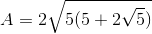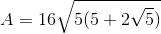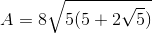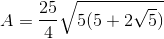Explanation:

The formula for the area of a regular pentagon is given by the equation: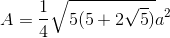where a is represented by the length of one side. Let's begin by finding the side length of the regular pentagon. If the perimeter is 40, then we can divide by 5 (the number of sides) to find a side length of 8.

If we plug in 8 into the equation: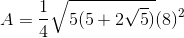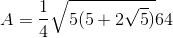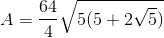### Example Question #2 : Pentagons

In a five-sided polygon, one angle measures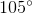. What are the possible measurements of the other angles?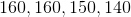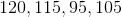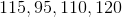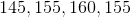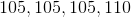Explanation:

To find the sum of the interior angles of any polygon, use the formula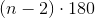, where n represents the number of sides of a polygon.

In this case: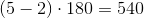The sum of the interior angles will be 540. Go through each answer choice and see which one adds up to 540 (including the original angle given in the problem).

The only one that does is 120, 115, 95, 105 and the original angle of 105.

### Example Question #51 : Geometry

In a particular heptagon (a seven-sided polygon) the sum of four equal interior angles, each equal todegrees, is equivalent to the sum of the remaining three interior angles.

Quantity A:Quantity B: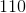The relationship cannot be determined.

The two quantities are equal.

Quantity B is greater

Quantity A is greater.

Quantity A is greater.

Explanation:

The sum of interior angles in a heptagon is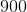degrees. Note that to find the sum of interior angles of any polygon, it is given by the formula: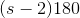degrees, whereis the number of sides of the polygon.

Three interior angles (call them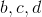)  are unknown, but we are told that the sum of them is equal to the sum of four other equivalent angles (which we'll designate):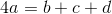Further more, all of these angles must sum up todegrees: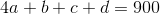We may not be able to find, or, indvidually, but the problem does not call for that, and we need only use their relation to, as stated in the first equation with them. Utilizing this in the second, we find: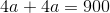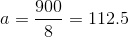### Example Question #1 : How To Find An Angle In A Pentagon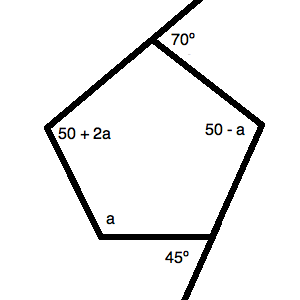What is the value ofin the figure above?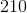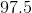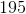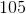Explanation:

Always begin working through problems like this by filling in all available information. We know that we can fill in two of the angles, giving us the following figure: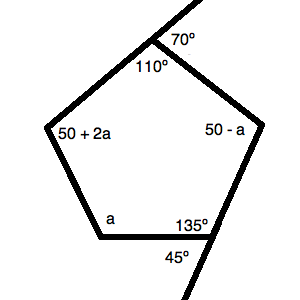Now, we know that for any polygon, the total number of degrees in the figure can be calculated by the equation: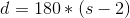, whereis the number of sides.

Thus, for our figure, we have: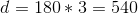Based on this, we know: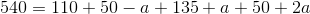Simplifying, we get: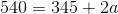Solving for, we get: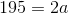or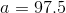### Example Question #1 : How To Find An Angle In A Pentagon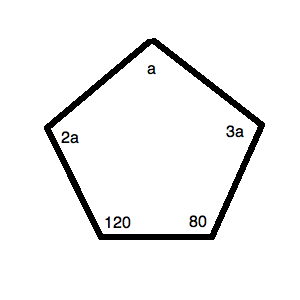Quantity A: The measure of the largest angle in the figure above.

Quantity B:Which of the following is true?

Quantity A is larger.

Quantity B is larger.

The two quantities are equal.

The relationship cannot be determined.

Quantity A is larger.

Explanation:To begin, recall that the total degrees in any figure can be calculated by:, whererepresents the total number of sides. Thus, we know for our figure that: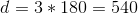Now, based on our figure, we can make the equation: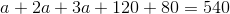Simplifying, we get: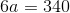or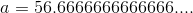This means that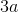is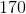. Quantity A is larger.

### All GRE Math Resources